Mathematics
Easy

Question

# In the inverse variation , x = -8 when y = -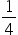. What is the value of y when x = 4 ?

##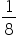-2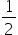-Hint:

## The correct answer is:### We have to find the value of y when x is 4.We have, y =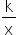=>-=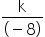=>k = 2.So, y =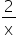=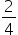=.Hence, the correct option is B.

In inverse variation, we have x1y= x2y2.

### Related Questions to study#### With Turito Foundation.#### Get an Expert Advice From Turito.